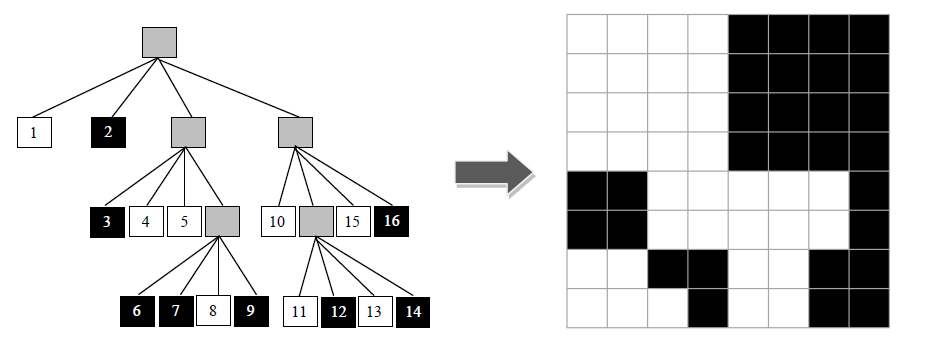A quadtree, first introduced by Finkel and Bentley, is a tree data-structure in which each internal node has exactly four children. Quadtrees are often used for problems that can be mapped into a two-dimensional space which is then recursively subdivided it into four equally-sized regions while a certain condition holds. The problem consists in reading a compressed binary image represented with a quadtree and determining which pixels are

set to white.

For a better understanding of this problem, consider the third test case from Sample Input, represented in the figure. The uncompressed binary image is composed by (8 x 8) pixels, where 35 of them are white. Notice each node in the quadtree is mapped into a square area from the target image. White nodes denote areas composed by white pixels exclusively, whereas black nodes denote areas with only black pixels; finally, gray nodes are composed by white and black pixels and thus, they need to be subdivided into four new square areas. Notice that the order of visiting square areas is: left to right and top to bottom.### 输入格式

The first line contains an integer N > 0 denoting the number of test cases.

The next N lines start each with the length L of the target image; L has to be a power of 2.

The length is followed by a space and a sequence of 0, 1 and *, denoting black, white and gray nodes of the quadtree, respectively. The quadtree is traversed in pre-order.

### 输出格式

The output consists of N lines containing each a comma-separated list of either:

a) (x,y) position of a pixel adjacent horizontally by black pixels, or

b) (xi-xf,y), where xf > xi: a sequence of white pixels at row y surrounded by black pixels.

The following holds: 1 <= x, xi, xf, y <= L. Traverse the binary image from left to right, top to bottom.

If L is not a power of 2, the output should display the text “Invalid length”, instead.

### 样例

Input
3
4 **1000*010010
7 *101*0100
8 *10*011*0010*1*101010

Output
(1,1),(4,1),(1-2,3),(1-2,4)
Invalid length
(1-4,1),(1-4,2),(1-4,3),(1-4,4),(3-7,5),(3-7,6),(1-2,7),(5-6,7),(1-3,8),(5-6,8)


22 人解决，24 人已尝试。

24 份提交通过，共有 43 份提交。

3.6 EMB 奖励。